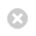Close

Mathematics

Home# Analysis

A lot of our research is concerned with the spectral theory (mostly of partial differential and difference equations). A significant proportion of our work concentrates on borderline areas between analysis and other fields, such as: geometry, number theory, mathematical physics and probability theory. More specifically, within our group we have people working in the following areas:

• Spectral geometry;
• Periodic and almost-periodic spectral problems;
• Toeplitz operators;
• Spectral theory of automorphic forms;
• Geometric scattering theory;
• Random operators;
• Matrix-valued operators (in particular, Dirac operators);
• Integrable systems;
• Nevanlinna Theory;
• Statistical Mechanics.

#### Group Members

Prof Rod Halburd, Professor of Mathematics
Professor of Mathematics
Professor of Mathematics